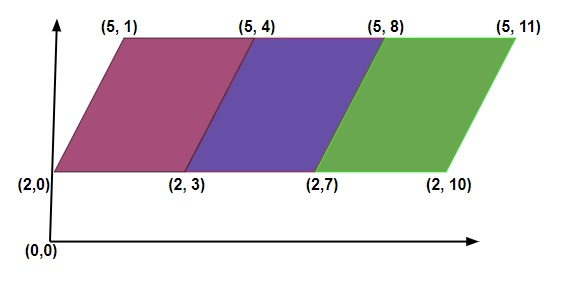# Count of parallelograms in a plane in C++

We are given a plane containing points forming the parallelogram and the task is to calculate the count of parallelograms that can be formed using the given points. In parallelograms opposite sides of a quadrilateral are parallel and therefore opposite angles are equal.

Input

int a[] = {0, 2, 5, 5, 2, 5, 2, 5, 2}
Int b[] = {0, 0, 1, 4, 3, 8, 7, 11, 10}Output − Count of parallelograms in a plane − 3

Explanation − we are given with the (x, y) points and using these points we can form a count of 3 parallelograms as shown in the figure.

Input

a[] = {0, 3, 1, 4, 1, 5}
b[] = {0, 1, 3, 4, 4, 4}

Output − Count of parallelograms in a plane − 1

Explanation − we are given with the (x, y) points and using these points we can form a count of 1 parallelogram as shown in the figure.

## Approach used in the below program is as follows

• Input array_1 for the x-coordinate values and array_2 for the y-coordinate values

• Calculate the size of array_1 and pass the data to the function for further processing

• Create a variable of type map that will form pair and store the integer type data

• Create a temporary variable count to store the total of parallelograms that can be formed

• Start loop FOR i from 0 till the size of an array_1

• Start another loop FOR j from i+1 till the size of an array_1

• Inside the loops, set a_mid with a[i] + a[j] and set b_mid with b[i] + b[j]

• And increment the mid values of array_1 and array_2 by 1

• Start another loop that will traverse the map from beginning till the end

• Inside the loop, set a temporary variable with the y value of the pair

• And set count with temp * (temp -1 ) / 2

• Return count

• Print the result.

## Example

Live Demo

#include <bits/stdc++.h>
using namespace std;
//Count of parallelograms in a plane
int parallelogram(int a[], int b[], int size){
map<pair<int, int>, int> um;
int count = 0;
for (int i=0; i<size; i++){
for (int j=i+1; j<size; j++){
int a_mid = a[i] + a[j];
int b_mid = b[i] + b[j];
um[make_pair(a_mid, b_mid)]++;
}
}
for (auto it = um.begin(); it != um.end(); it++){
int temp = it->second;
count+= temp*(temp - 1)/2;
}
return count;
}
int main(){
int a[] = {0, 3, 1, 4, 1, 5};
int b[] = {0, 1, 3, 4, 4, 4};
int size = sizeof(a) / sizeof(int);
cout<<"Count of parallelograms in a plane: "<<parallelogram(a, b, size) << endl;
return 0;
}

## Output

If we run the above code it will generate the following output −

Count of parallelograms in a plane: 1

Updated on: 31-Aug-2020

54 Views